# Java Program to Get User Input and Print on Screen

In this tutorial you will learn about the Java Program to Get User Input and Print on Screen and its application with practical example.

## Java Program to Get User Input and Print on Screen

In this tutorial, we will learn to create a Java program that will get user input and print on screen in Java programming.

## Prerequisites

Before starting with this tutorial, we assume that you are the best aware of the following Java programming topics:

• Operators in Java Programming.
• Basic Input and Output function in Java Programming.
• Basic Java programming.

## What is a data type?

A data type, in every programming language, specifies which type of value a variable is going to hold in the program. i.e. integer, character, float, double.

## Program Print output to the user.

In this tutorial, First, we will take input from the user in number format using a scanner class object in the program. Then we will store it in a variable in the memory. Then we will print that number to the user using the print function.

## Output:-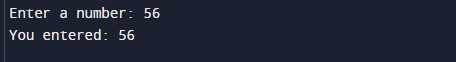In the above program, we have first initialized the required variable.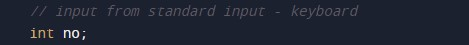• no = it will hold the input integer value from the user.

Importing the scanner in util package for the program.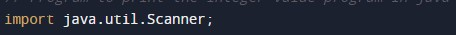Taking the input from the user in integer format.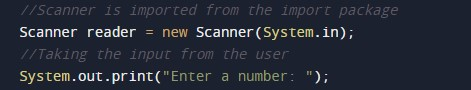Printing the integer value to the user by print function.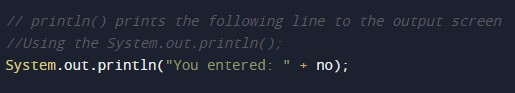In this tutorial we have learn about the Java Program to Get User Input and Print on Screen and its application with practical example. I hope you will like this tutorial.